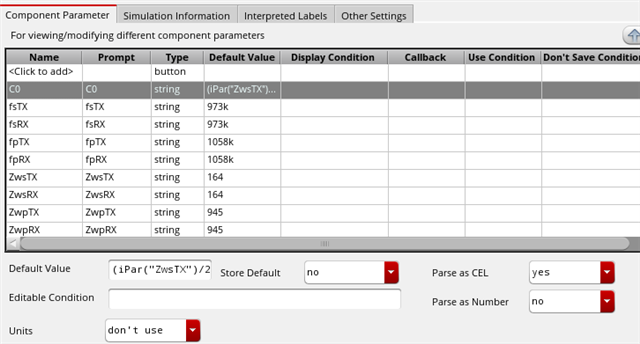CommunityForums Custom IC Design variables from CDF parameters

variables from CDF parameters

Hello, I have a cell in which the parameters of each instance depend from some CDF parameters of the cell itself.

However the formulas are extremely long, and some instances should refer to the value of different instances.

Example: C1 capacitance is a function of the capacitane of C2, which is defined out of CDF parameters.

Is there a way to refer to C2 within C1? Like an iPar of a different instance.

Pasting the formula used in C2 is of course possible, however the additional operations would increase the length even more.

Alternatively, is there anything like a "hidden" CDF parameter, defined out of other CDF parameters?

• You can't refer to another instance in CDF parameter expressions. You could however create a CDF parameter for this cell and have that marked as not displayed, and then use pPar() to reference this common parameter - not sure if that's what you mean?

Andrew

• Clear, it's not possible to refer to another instance.

In the alternative I meant to use some kind of intermediate variable that coul be referred to by all instances.

For example:

variable1=Ppar("p1")**2 / Ppar("p2")

instance1 capacitance:  Ppar("p3")**2 - variable1

instance2 capacitance:  Ppar("p4") - variable1

.....

But of course it's possible to paste the definition of variable1 into the instances, that's how I do it; only the strings are extremely long and prone to typing errors.

• You could do this by having another CDF parameter (variable1) on the containing cell and then that could be defined as:

variable1: iPar("p1")**2'iPar("p2")

and then:

instance1 capacitance: pPar("p3")**2-pPar("variable1")
instance2 capacitance: pPar("p4")-pPar("variable1")

Andrew

• Thanks, that's what I was looking for!

In principle it works, but somehow there is a limitation in the formula that I specify in the CDF form.For example:

iPar("ZwsTX") + iPar("fpTX")

works, while adding a division does not (even within brackets):

iPar("ZwsTX")/2 + iPar("fpTX")

same for sqrt or power (**)

Any idea if there is a special syntax to follow?

• That should work - I see no reason why it would not.

Related